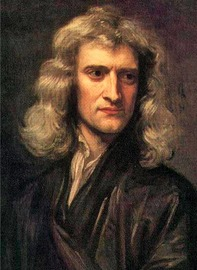On this day many years ago, a baby was born who would grow up to change the course of human history
This December 25th marks the 377th anniversary of the birth of Sir Isaac Newton.

Here is a Newtonmass Carol to celebrate the occasion (sung to the tune of “God Rest ye Merry, Gentlemen”):

Oh, rest ye merry, physicists
Let nothing you dismay.
Remember Isaac Newton
was born on Christmas Day!
His gravity and calculus and “f” equals “m” “a”!
Oh, pillars of physics and math, physics and math,
Oh, pillars of physics and math!

A factor of big G – the same
for flea and giant star.
Then multiply the masses
and divide by square of “r”.
The force that keeps us on the earth
and orbits moons afar!
Oh, pillars of physics and math, physics and math,
Oh, pillars of physics and math!

Now, calculus is math for those
who change things bit by bit.
To figure out derivatives
and get the curve to fit.
Then integrate and you can find the area under it!
Oh, pillars of physics and math, physics and math,
Oh, pillars of physics and math!

Sir Isaac took a beam of light
and passed it through some glass.
“What shall I call these colours?”
was the question he did ask.
And now we live with ROY G BIV
in every optics class.
Oh, pillars of physics and math, physics and math,
Oh, pillars of physics and math!

We use 3 laws of motion to
put rockets in the sky.
The first one says inertia will
explain the reason why.
An object, stopped, won’t move unless
a motive force comes by.
Oh, pillars of physics and math, physics and math,
Oh, pillars of physics and math!

The second law of motion gives us
all a balance sheet
Describing what will happen when
perchance two objects meet.
The action and reaction
Are the same and opposite
Oh, pillars of physics and math, physics and math,
Oh, pillars of physics and math!

Now, when a force acts on a mass,
it will accelerate.
The third law tells us why
Our mass is not the same as weight.
And so we see that objects to each other gravitate.
Oh, pillars of physics and math, physics and math,
Oh, pillars of physics and math!

Oh, rest ye merry, physicists
Let nothing you dismay.
Remember Isaac Newton
was born on Christmas Day!
His gravity and calculus and “f” equals “m” “a”!
Oh, pillars of physics and math, physics and math,
Oh, pillars of physics and math!(c) Seanna & Stephen Watson, Dec 2014. Free to use or distribute, with attribution.